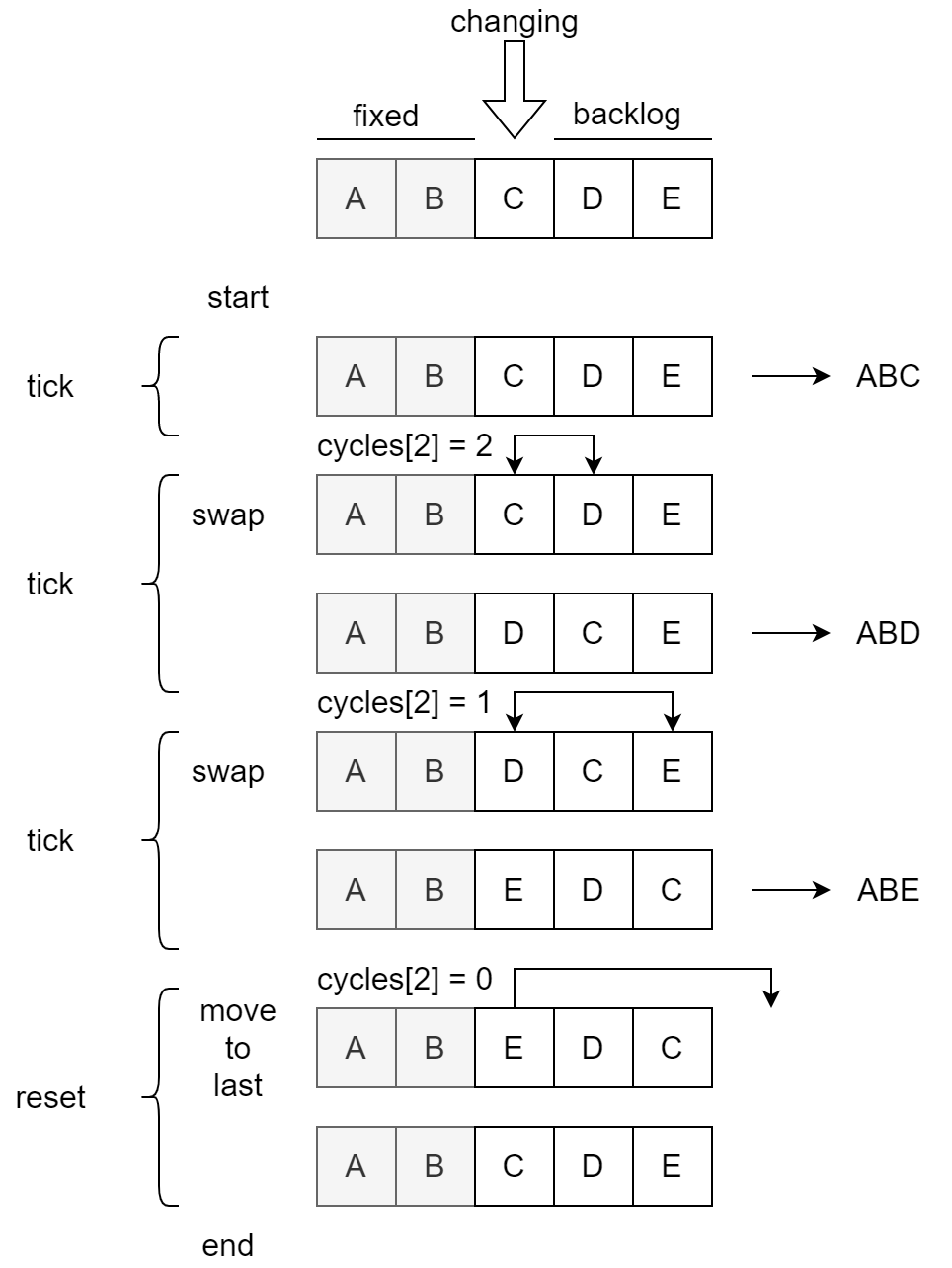# Python `itertools.permutations` 使用的排列算法

##### May 10, 2022 - 算法

TL;DR：这本质上是一个基于回溯，利用元素交换的递归排列生成算法，但被重写成了循环形式（可能出于效率考量）。

## 引子

``````>>> list(permutations('ABCD', 2))
[('A', 'B'), ('A', 'C'), ('A', 'D'),
('B', 'A'), ('B', 'C'), ('B', 'D'),
('C', 'A'), ('C', 'B'), ('C', 'D'),
('D', 'A'), ('D', 'B'), ('D', 'C')]
``````

``````def permutations(iterable, r=None):
# permutations('ABCD', 2) --> AB AC AD BA BC BD CA CB CD DA DB DC
# permutations(range(3)) --> 012 021 102 120 201 210
pool = tuple(iterable)
n = len(pool)
r = n if r is None else r
if r > n:
return
indices = list(range(n))
cycles = list(range(n, n-r, -1))
yield tuple(pool[i] for i in indices[:r])
while n:
for i in reversed(range(r)):
cycles[i] -= 1
if cycles[i] == 0:
indices[i:] = indices[i+1:] + indices[i:i+1]
cycles[i] = n - i
else:
j = cycles[i]
indices[i], indices[-j] = indices[-j], indices[i]
yield tuple(pool[i] for i in indices[:r])
break
else:
return
``````

### 寻找相关信息

• Stack Overflow 的答主 Alex 写了一篇长文回答，但一开头就说「你需要理解 Permutation Cycle 的数学理论，才能理解这个算法」。于是我又回头恶补了一番组合数学，然而就算我大概知道 Permutation Cycle 是怎么一回事，对这个算法依然毫无头绪。（评论中也有人自称有 6 年抽象代数经验，但不认为这个算法与数学相关。）不过 Alex 的回答提供了一些有用的直觉，至少读完这个回答我大概理解 `cycles` 在算法执行过程中是如何变化的了。具体而言，`cycles` 的变化类似于「倒计时」，或者说「带借位的减法」，每次最后一位到 0 时，就会触发前面的一位减 1。然而我对`indices` 的变化依然云里雾里。
• 知乎提问中，答主「杨个毛」提供了一个看起来很有说服力的回答（剧透：而且也的确如此）：「那个代码可以看成下列递归程序的强行非递归版」，指出了 `cycles`变进制数，并提供了一个原始递归版本。可惜的是，对于这个算法为什么可以输出正确结果依然没有解释（也有可能是我没有完全理解）。

## 算法

### 起步

• `n` 代表输入 iterable 的长度
• `r` 代表输出的排列元组的长度

• 对输入的 `iterable` 会遍历其所有元素并放在 `pool`
• 每次算法输出结果（`yield`），实际上只是取了 `indices` 列表的前 `r` 个 index，并输出 `pool` 中的对应元素。

1. 理解 `cycles` 的变化
2. 理解 `indices` 的变化，并尝试说明这个算法的正确性
3. 尝试重新实现这个算法的「原始」递归版本

### `cycles`

``````[4,3] -> [4,2] -> [4,1] -> {4,0} -> {4,3} ->
[3,3] -> [3,2] -> [3,1] -> {3,0} -> {3,3} ->
[2,3] -> [2,2] -> [2,1] -> {2,0} -> {2,3} ->
[1,3] -> [1,2] -> [1,1] -> {1,0} -> {1,3} -> {0,3} -> {4,3}
``````

• 一开始 `cycles` 被初始化为 4，`cycles` 被初始化为 3。
• 之后的循环中，一般是 `cycles` 不断被减 1。当 `cycles != 0` 时，算法会产生一次输出。
• 如果 `cycles == 0`，会导致 `cycles` （前一位）减 1，并将 `cycles` 重设回其初始值 3。
• 最后当 `cycles == 0` 时，算法结束。

• 一开始 `cycles` 被初始化为 `range(n, n-r, -1)`，即 `cycles=n``cycles=n-1`，…，`cycles[i]=n-i`
• 之后的循环中，通常只有最后一个元素 `cycles[r-1]` 在不断递减。如果 `cycles[r-1]` 递减后值不为 0，则算法会产生一次输出。用我们之前提到的「倒计时」类比，可以将这种情况称之为 tick。
• 如果 `cycles` 中的某个元素（如 `cycles[i]`）为 0，会导致其前面的元素被（`cycles[i-1]`）减 1，并将这个元素（`cycles[i]`）重设回其初始值（`n-i`）。这一行为类似于倒计时中秒为 0 时会导致分减 1，并将秒重设回 0。继续用「倒计时」类比，可以将这种情况称之为 reset。

• 一开始 `cycles` 被初始化为 `range(n, n-r, -1)`，而可以计算出对给定的 `n`, `r` 所有的排列有 `P(n,r) = n*(n-1)*...*(n-r-1)` 种。
• 每次 tick，算法生成一个排列，消耗一个选择，`cycles[i]` 也减 1
• 每次 reset，实际上就是在 `cycles` 这个变进制数上的借位减法
• 最后在 `cycles` 上首位为 0 时算法结束，代表所有排列的选择都已经被消耗（输出）了，已经没有更多的排列了。

### `indices`• 图的阅读顺序是从上至下，按照时间顺序展示了`indices` 列表的变化。左侧为各阶段具体行为的标注，右侧为算法输出。
• 这里选择展示前 3 次输出（即 `i=2`），对应 `cycles` 从 3 到 0 的过程。别忘了每次进入循环前 `cycles` 会被递减。
• 为方便描述，我们可以把 `indices` 列表拆成 3 个部分：
• fixed：`indices[0:1]`，在执行时不会变化
• changing：`indices`，这是在不断被交换的元素，和 fixed 一起组成了算法输出（`indices[0:2]`
• backlog：`indices[3:4]`，这里存放着已经被使用的元素和还未被使用的元素
• 在每个 tick 中，changing 位置上的元素和 backlog 中的一个元素交换（`swap`），并触发一次输出。可以注意到，交换前后 backlog 中元素依然维持其相对顺序。
• ABC{DE} -> ABD{CE} -> ABE{CD}
• 注：这里将算法开始时的首次输出也视作一个 tick，因为进入循环前依然触发了递减，消耗了一个可能性。
• 相关代码：`indices[i], indices[-j] = indices[-j], indices[i]`
• 算法结束前，一共触发了 3 次输出。这已经穷尽了固定前缀为 `AB`，最后一个可选项为 `C`, `D`, `E` 的排列。
• 在最后 reset 时，changing 位置上的元素被移到了 backlog 后（`move_to_last`）。这一步完成后，changing + backlog 这个子列表（`indices[2:4]`）恢复了和算法开始时一样的顺序。
• AB{E}CD -> ABCD{E}
• 相关代码：`indices[i:] = indices[i+1:] + indices[i:i+1]`

• 分步解决一个问题，每步中固定之前步骤的选择不变
• 算法执行中，遍历当前步的所有可能性
• 算法执行后，取消当前步的计算，退回上一步，选择下一个可选项

### 重新实现

• 递归调用时，需要一个参数指定当前修改的元素（changing）的 index
• 在每一层调用中，交换 changing 和 backlog 中的所有项，并在每次交换（做出选择）后触发下一层
• 如果 backlog 中的所有可选项都已经被选择（消耗）了，就把当前的 changing 移到 backlog 后，以撤销这一层的选择

``````## a reimplementation of `itertools.permutation`

# helpers
def swap(list, i, j):
list[i], list[j] = list[j], list[i]

def move_to_last(list, i):
list[i:] = list[i+1:] + [list[i]]

def print_first_n_element(list, n):
print("".join(list[:n]))

# backtracking dfs
def permutations(list, r, changing_index):
if changing_index == r:
# we've reached the deepest level
print_first_n_element(list, r)
return

# a pseudo `tick`
# process initial permutation
# which is just doing nothing (using the initial value)
permutations(list, r, changing_index + 1)

# note: initial permutaion has been outputed, thus the minus 1
remaining_choices = len(list) - 1 - changing_index
# for (i=1;i<=remaining_choices;i++)
for i in range(1, remaining_choices+1):
# `tick` phases

# make one swap
swap_idx = changing_index + i
swap(list, changing_index, swap_idx)
# finished one move at current level, now go deeper
permutations(list, r, changing_index + 1)

# `reset` phase
move_to_last(list, changing_index)

# wrapper
def permutations_wrapper(list, r):
permutations(list, r, 0)

# main
if __name__ == "__main__":
my_list = ["A", "B", "C", "D"]
permutations_wrapper(my_list, 2)
``````

### 递归转循环优化

• `r` 是已知且固定的，即栈的最大深度为 `r`，因此可以用一个固定大小的列表来表示栈中（每一层递归）的状态。

• `changing_idx` 是每层递归的输入，代表当前层变化的元素。`changing_idx` 可以从栈的深度计算出来，栈底为 0，再上一层为 1… 因此实际上不需要维护。

• `i``swap_idx` 为下一次交换的目标元素 index。这无法从栈本身的信息计算得到，需要我们维护。

• 进入或重新回到当前层递归的开始位置，可以用 `i``swap_idx` 推算得到：如果还有剩余可选项，则跳转到 tick；反之跳转到 reset

• 长度固定为 `r`
• 每个元素上维护下一次交换的目标元素 index